Published: 31 December 2016

# A Unified method for vibration analysis of moderately thick annular, circular plates and their sector counterparts subjected to arbitrary boundary conditions

Dongyan Shi2
Anees Ur Rehman3
Hafiz M. Waqas4
1, 2, 3, 4College of Mechanical and Electrical Engineering, Harbin Engineering University, Harbin, China
Corresponding Author:
Views 70

#### Abstract

The vibrations of circular, annular and sector plates are different boundary value problems due to different edge conditions and thus have been treated separately using different solution algorithms and procedures. In this paper, a unified method is proposed for vibration analysis of moderately thick annular, circular plates and their sector counterparts with arbitrary boundary conditions. The unification of these plates is physically achieved by applying the coupling spring’s technique at the radial edges to ensure appropriate continuity conditions. Irrespective of the shape of the plate and the type of boundary conditions, each of the displacement function is expressed as a new form of trigonometric expansion with high convergence rate. Unlike most of the previous studies the current method can be universally applied to a wide range of vibration problems involving different shapes, boundary conditions, varying materials and geometric properties without modifying the solution algorithms and procedure. Furthermore, the current method can easily be applied to sector plates with an arbitrary inclusion angle of 2$\pi$. The accuracy, reliability and versatility of the proposed method are fully demonstrated with several numerical examples for different shapes of plates and under different boundary conditions.

## 1. Introduction

Circular, annular and their sectorial counterparts are important structural components widely used in many engineering fields like civil, mechanical and marine engineering. As far as previous literature is concerned different solution algorithms and procedures have been adopted to study their vibration characteristics. The main reason behind these different solution algorithms and procedures was difference in their geometries resulting in different edge conditions.

A lot of research work has been done to study their dynamic characteristics under different boundary conditions. The important and comprehensive review on this subject can be found in Leissa’s 1973 book. The initial study on vibrations of circular plates or disks was done by Deresiewicz and Mindlin . Employing the classical thin plate theory and Mindlin plate theory, these two researchers studied the vibration characteristics of axially symmetric circular disks. This work was further extended by Soni et al.  to axisymmetric orthotropic non uniform circular discs. They carried out their research using the same Mindlin plate theory and Chebyshev collocation technique. This technique was later employed by Gupta et al. to polar orthotropic annular Mindlin plates with non-uniform thickness . Using Finite Element Method and three-dimensional finite strip model, Cheung et.al studied the vibration characteristics of thick and thin sector plates subjected to different types of classical boundary conditions [4, 5]. Investigation on vibration characteristics of annular sector plates having internal radial line and circumferential arc supports was carried out by Xiang et al. [6-7]. In another study Xiang et al. used first order shear deformation theory and studied the vibration response of thick circular and annular plates with internal ring stiffeners . Later he extended his research to stepped circular Mindlin plates by employing domain decomposition technique to study the vibration characteristics . Another similar study was performed by B. Singh and S. M. Hassan . They studied the out of plane vibrations of a circular plate with different thickness variation. They approximated the thickness polynomial by interpolating the sample points along the thickness of the plate. In another study a combination of Rayleigh-Ritz method and Lagrange multiplier method was developed by S. Kitipornchai et al. to study the vibration characteristics of arbitrary shaped plates with corner supports . Exact solution for annular sector plates subjected to simply supported radial edge conditions and general boundary conditions at circular edges was obtained by McGee et al.  employing the Mindlin plate theory and using ordinary and modified Bessel functions of the first and second kind.

Differential quadrature method was employed by various researchers to study the vibration characteristics of sector plates, annular sector plates and solid circular plates. Extensive results were reported for these plates subjected to various sets of classical boundary conditions [13-15]. Huang et al.  employed Frobenius method on orthotropic sector plates and studied the effect of Young modulus and shear modulus on the vibration characteristics of these plates. In another important research on thick circular and annular plates with uniform, linear and quadratic change in thickness along the radial edge was performed by Jae Hoon Kang . A similar three-dimensional study of thick annular and circular plates was carried out by J. So et al.  employing Rayleigh-Ritz method. In their research they used trigonometric functions and algebraic polynomial as admissible displacement functions along the circumferential and radial and axial coordinates respectively. Another three-dimensional study of annular and circular plates was performed by Zhou et.al. They employed Chebyshev-Ritz technique and used Chebyshev polynomial as admissible function. Later they extended the same Chebyshev-Ritz technique to annular sector plates [19, 20]. Another important three-dimensional investigation on annular plates resting on elastic foundation was done by Hashemi et al. They used polynomial-Ritz approach and studied the effect of cutout ratio, thickness to radius ratio and elastic foundation on the vibration characteristics of annular plates subjected to various combinations of classical boundary conditions .

Discrete singular convolution method was used by Civalek et.al to investigate the vibration characteristics of Mindlin annular plates and thick circular plates [22, 23]. Similarly employing the Mindlin plate theory and first order shear deformation theory, Jomehzadeh et.al investigated the transverse vibrations of isotropic sector plate and moderately thick annular sector plates subjected to simply supported boundary conditions and arbitrary boundary conditions at radial and circular edges respectively [24-25]. In plane free vibration analysis of isotropic homogeneous circular disks subjected to arbitrary boundary conditions at the inner and outer edges was investigated by Bashmal et al. by employing two-dimensional linear plane stress theory. In another study he employed Rayleigh-Ritz method to study the vibration characteristics of annular disk with point elastic support [26, 27]. Similarly, Ravari et al. investigated the in plane vibrations of orthotropic circular annular plates by using Helmholtz decomposition technique and separation of variables method .

In other similar studies on circular, annular and sector plates, Sari et al.  used Chebyshev collocation method to study the vibration characteristics of Mindlin annular plates with damaged boundary conditions. Similarly, Reddy’s higher order shear deformation theory was employed by Bisadi et.al and Es’Haghi [30, 31] to investigate the vibration characteristics of thick circular and annular plates subjected to different combinations of classical boundary conditions at edges. Employing the boundary restraining springs technique Shi et.al proposed a generalized Fourier series method to study the annular sector plates subjected to elastic boundary conditions at each edge [32-33]. Later X. Shi et al.  proposed a unified method for vibration analysis of circular, annular and their sector counterparts by employing coupling springs technique at the coupling edge. The same idea has been adopted here to develop a unified method to study the vibration characteristics of Mindlin circular, annular and their sector counter parts subjected to general elastic boundary conditions. The beauty of this method is that it does not require any modification in the procedure or solution algorithm to accommodate these different geometries and boundary conditions.

## 2. Theoretical formulation

### 2.1. Description of the model

Consider a moderately thick annular sector plate with internal radius $a$, outer radius $b$, thickness $h$ and width $R$ in the radial direction as shown in Fig. 1. The angle $\varphi$ represents the sector angle of the plate. The plate geometry and dimensions are defined in the cylindrical coordinate system $\left(r,\varphi ,z\right)$.

Fig. 1Geometry of moderately thick annular sector plate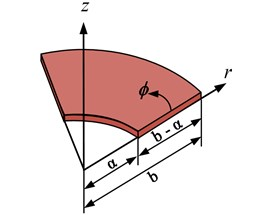a)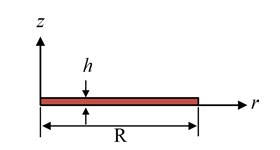b)

The elastic boundary conditions along the edges are specified using boundary spring technique. One translational and two rotational springs of arbitrary stiffness values are attached at each edge to simulate arbitrary boundary conditions. All the classical sets of boundary conditions can easily be achieved by varying the stiffness value of each spring from zero to an infinitely large number i.e. 1014. It can be seen in Fig. 2 that an annular plate can be obtained by annular sector plate when the sector angle becomes equal to 2$\pi$, a circular sector plate can be obtained from annular sector plate if the inner radius $a$ becomes equal to 0. Similarly, a circular plate can be obtained when the inclusion angle of the annular sector plate becomes equal to 2$\pi$ and the inner radius $a$ also becomes equal to 0. Therefore, the solution algorithm and procedure will be developed in such a way that it can easily be applied to annular, circular and circular sector plates just by varying geometric parameters mentioned earlier.

Fig. 2a) Annular sector plate, b) annular plate, c) circular sector plate, d) circular plate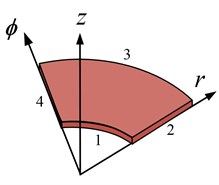a)$0\le \varphi <2\pi$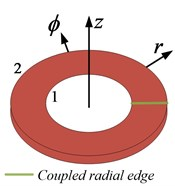b)$\varphi =2\pi$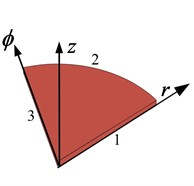c)$0\le \varphi <2\pi$, $\alpha =0$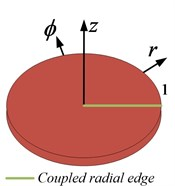d)$\varphi =2\pi$, $\alpha =0$

### 2.2. Formulation

In the framework of first order shear deformation plate theory, the displacement field in an arbitrary point of a moderately thick annular sector pate is given by:

1
${u}_{r}\left(r,\varphi ,z,t\right)={u}_{r}\left(r,\varphi ,z\right)+z{\theta }_{r}\left(r,\varphi ,t\right),$
${u}_{\varphi }\left(r,\varphi ,z,t\right)={u}_{\varphi }\left(r,\varphi ,z\right)+z{\theta }_{\varphi }\left(r,\varphi ,t\right),$
$w\left(r,\varphi ,z,t\right)={w}_{o}\left(r,\varphi ,t\right),$

where ${\theta }_{r}$ and ${\theta }_{\varphi }$ represents the rotation of transverse normal with respect to $\varphi$ and $r$ directions, $z$ is the thickness coordinate, ${u}_{r}$ and ${u}_{\varphi }$ are displacements of the mid plane in $r$ and $\varphi$ directions, respectively, ${w}_{o}$ is the transverse displacement and $t$ is the time. Thus the corresponding strains at this point are defined in terms of middle surface strains, curvature and twist changes as:

2
${\epsilon }_{r}={\epsilon }_{r}^{o}+z{\chi }_{r},{\epsilon }_{\varphi }={\epsilon }_{\varphi }^{o}+z{\chi }_{\varphi },{\epsilon }_{z}=0,$
${\gamma }_{r\varphi }={\gamma }_{r\varphi }^{o}+z{\chi }_{r\varphi },{\gamma }_{rz}={\gamma }_{rz}^{o},{\gamma }_{\varphi z}={\gamma }_{\varphi z}^{o},$

where the middle surface strains, curvature and twist changes are written as:

3
${\epsilon }_{r}^{o}=\frac{\partial {u}_{r}}{\partial r},{\chi }_{r}=\frac{\partial {\theta }_{r}}{\partial r},$
${\epsilon }_{\varphi }^{o}=\frac{\partial {u}_{\varphi }}{r\partial \varphi }+\frac{{u}_{r}}{r},{\chi }_{\varphi }=\frac{\partial {\theta }_{\varphi }}{r\partial \varphi }+\frac{{\theta }_{r}}{r},$
${\gamma }_{r\varphi }^{o}=\frac{\partial {u}_{\varphi }}{\partial r}+\frac{\partial {u}_{r}}{r\partial \varphi }-\frac{{u}_{\varphi }}{r},{\chi }_{r\varphi }=\frac{\partial {\theta }_{\varphi }}{\partial r}+\frac{\partial {\theta }_{r}}{r\partial \varphi }-\frac{{\theta }_{\varphi }}{r},$
${\gamma }_{rz}^{o}=\frac{\partial {w}_{o}}{\partial r}+{\theta }_{r},{\gamma }_{\varphi z}^{o}=\frac{\partial {w}_{o}}{r\partial \varphi }+{\theta }_{\varphi }.$

Assuming the plain stress distribution in accordance with Hooks law, the stress resultants are obtained for Mindlin annular plate by integrating the stresses as shown below:

4
${M}_{r}=\underset{-h/2}{\overset{h/2}{\int }}{\sigma }_{r}zdz=D\left[\frac{\partial {\theta }_{r}}{\partial r}+\frac{\nu }{r}\left({\theta }_{r}+\frac{\partial {\theta }_{\varphi }}{\partial \varphi }\right)\right],$
${M}_{\varphi }=\underset{-h/2}{\overset{h/2}{\int }}{\sigma }_{\varphi }zdz=D\left[\frac{1}{r}\left({\theta }_{r}+\frac{\partial {\theta }_{\varphi }}{\partial \varphi }\right)+\nu \left(\frac{\partial {\theta }_{r}}{\partial r}\right)\right],$
${M}_{r\varphi }=\underset{-h/2}{\overset{h/2}{\int }}{\tau }_{r\varphi }zdz=D\left(\frac{1-\nu }{2}\right)\left[\frac{1}{r}\left(\frac{\partial {\theta }_{r}}{\partial \varphi }-{\theta }_{\varphi }\right)+\frac{\partial {\theta }_{\varphi }}{\partial r}\right],$
${Q}_{r}={K}^{2}\underset{-h/2}{\overset{h/2}{\int }}{\tau }_{rz}dz={K}^{2}Gh\left[{\theta }_{r}+\frac{\partial {w}_{o}}{\partial r}\right],$
${Q}_{\varphi }={K}^{2}\underset{-h/2}{\overset{h/2}{\int }}{\tau }_{\varphi z}dz={K}^{2}Gh\left[{\theta }_{\varphi }+\frac{1}{r}\frac{\partial {w}_{o}}{\partial \varphi }\right],$

where ${M}_{r}$, ${M}_{\varphi }$ and ${M}_{r\varphi }$ are the bending moments per unit length of the plate, ${Q}_{r}$ and ${Q}_{\varphi }$ are the transverse shear forces per unit length of the plate, ${\sigma }_{r}$, ${\sigma }_{\varphi }$ are the normal stresses, ${\tau }_{r\varphi }$, ${\tau }_{rz}$ and ${\tau }_{\varphi z}$ are the shear stresses, $h$ is the plate thickness, $E$ is the modulus of elasticity, $G=E/2\left(1+\nu \right)$ is the shear modulus, $\nu$ is the Poisson ratio, $D=E{h}^{3}/12\left(1-{\nu }^{2}\right)$ is the flexural rigidity and ${K}^{2}={\pi }^{2}/12$ is the shear correction factor to compensate for the error in assuming the constant shear stress throughout the plate thickness. The equation of motion of the Mindlin annular sector plate is given by:

5
$\frac{\partial {M}_{r}}{\partial r}+\frac{1}{r}\frac{\partial {M}_{r\varphi }}{\partial \varphi }+\frac{1}{r}\left({M}_{r}-{M}_{\varphi }\right)-{Q}_{r}=\frac{\rho {h}^{3}}{12}\left(\frac{{\partial }^{2}{\theta }_{r}}{\partial {t}^{2}}\right),$
$\frac{\partial {M}_{r\varphi }}{\partial r}+\frac{1}{r}\frac{\partial {M}_{\varphi }}{\partial \varphi }+\frac{2}{r}{M}_{r\varphi }-{Q}_{\varphi }=\frac{\rho {h}^{3}}{12}\left(\frac{{\partial }^{2}{\theta }_{\varphi }}{\partial {t}^{2}}\right),$
$\frac{\partial {Q}_{r}}{\partial r}+\frac{1}{r}\frac{\partial {Q}_{\varphi }}{\partial \varphi }+\frac{{Q}_{r}}{r}=\rho h\frac{{\partial }^{2}{w}_{o}}{\partial {t}^{2}}.$

The boundary conditions for an elastically restrained moderately thick annular sector plate are:

6
${k}_{a}{w}_{o}={Q}_{r},\mathrm{}\mathrm{}\mathrm{}\mathrm{}{K}_{a}^{r}{\theta }_{r}=-{M}_{r},\mathrm{}\mathrm{}\mathrm{}\mathrm{}{K}_{a}^{t}{\theta }_{\varphi }=-{M}_{r\varphi },\mathrm{}\mathrm{}\mathrm{}\mathrm{}r=a,$
${k}_{b}{w}_{o}=-{Q}_{r},\mathrm{}\mathrm{}\mathrm{}\mathrm{}{K}_{b}^{r}{\theta }_{r}={M}_{r},\mathrm{}\mathrm{}\mathrm{}\mathrm{}{K}_{b}^{t}{\theta }_{\varphi }={M}_{r\varphi },\mathrm{}\mathrm{}\mathrm{}\mathrm{}r=b,$
${k}_{\varphi 0}{w}_{o}=-{Q}_{\varphi },\mathrm{}\mathrm{}\mathrm{}\mathrm{}{K}_{\varphi 0}^{r}{\theta }_{\varphi }={M}_{\varphi },\mathrm{}\mathrm{}\mathrm{}\mathrm{}{K}_{\varphi 0}^{t}{\theta }_{\varphi }={M}_{r\varphi },\mathrm{}\mathrm{}\mathrm{}\mathrm{}\varphi =0,$
${k}_{\varphi 1}{w}_{o}={Q}_{\varphi },\mathrm{}\mathrm{}\mathrm{}\mathrm{}{K}_{\varphi 1}^{r}{\theta }_{\varphi }=-{M}_{\varphi },\mathrm{}\mathrm{}\mathrm{}\mathrm{}{K}_{\varphi 1}^{t}{\theta }_{\varphi }=-{M}_{r\varphi },\mathrm{}\mathrm{}\mathrm{}\mathrm{}\varphi =\alpha ,$

where ${k}_{a}$, ${k}_{b}$ (${k}_{\varphi 0}$ and ${k}_{\varphi 1}\right)$ are translational spring constants, ${K}_{a}^{r}$, ${K}_{b}^{r}$ (${K}_{\varphi 0}^{r}$ and ${K}_{\varphi 1}^{r}\right)$ are rotational spring constants attached in radial direction and ${K}_{a}^{t}$, ${K}_{b}^{t}$ (${K}_{\varphi 0}^{t}$ and ${K}_{\varphi 1}^{t}\right)$ are rotational spring constants attached in circumferential direction at $r=a$ and $b$ ($\varphi =0$ and $\varphi =\alpha \right)$ respectively. All the classical homogeneous boundary conditions can be simply considered as special cases when the spring constants are either extremely large or substantially small. For instance, a clamped boundary (C) is achieved by simply setting the stiffness of the entire springs equal to infinity (which is represented by a very large number, 1014). Inversely, a free boundary (F) is gained by setting the stiffness of the entire springs equal to zero. The units for the translational and rotational springs are N/m and Nm/rad, respectively.

### 2.3. Trigonometric series representation for the displacement functions

Regardless of the plate shape and type of boundary conditions, the displacement and rotation functions are invariably expressed in the form of simple trigonometric series expansion as:

7
${\theta }_{r\left(r,\varphi \right)}=\sum _{m=n=-2}^{\mathrm{\infty }}{A}_{mn}{\phi }_{m}\left(r\right){\phi }_{n}\left(\varphi \right),$
${\theta }_{\varphi \left(r,\varphi \right)}=\sum _{m=n=-2}^{\mathrm{\infty }}{B}_{mn}{\phi }_{m}\left(r\right){\phi }_{n}\left(\varphi \right),$
${w}_{o\left(r,\varphi \right)}=\sum _{m=n=-2}^{\mathrm{\infty }}{C}_{mn}{\phi }_{m}\left(r\right){\phi }_{n}\left(\varphi \right),$

where ${A}_{mn}$, ${B}_{mn}$, ${C}_{mn}$ denotes the expansion coefficients and:

${\phi }_{m}\left(r\right)=\left\{\begin{array}{l}\mathrm{c}\mathrm{o}\mathrm{s}{\lambda }_{m}\left(r\right),\mathrm{}\mathrm{}\mathrm{}\mathrm{}m\ge 0,\\ \mathrm{s}\mathrm{i}\mathrm{n}{\lambda }_{m}\left(r\right),\mathrm{}\mathrm{}\mathrm{}\mathrm{}m<0,\end{array}\right\{\phi }_{n}\left(\varphi \right)=\left\{\begin{array}{l}\mathrm{c}\mathrm{o}\mathrm{s}{\lambda }_{n}\left(\varphi \right),\mathrm{}\mathrm{}\mathrm{}\mathrm{}n\ge 0,\\ \mathrm{s}\mathrm{i}\mathrm{n}{\lambda }_{n}\left(\varphi \right),\mathrm{}\mathrm{}\mathrm{}\mathrm{}n<0,\end{array}\right\{\lambda }_{m}=\frac{m\pi }{R},\mathrm{}\mathrm{}\mathrm{}\mathrm{}{\lambda }_{n}=\frac{n\pi }{\alpha }.$

A solution can be obtained either in strong form by letting the series satisfy the relevant equations exactly on a point-wise basis, or in weak form by solving the series coefficients approximately using, for instance, the Rayleigh-Ritz technique. The weak form of solution will be sought here since it will be more attractive in modeling complex structures. To employ this method for this analysis, it is necessary to state the potential and kinetic energy in terms of displacement fields. The total potential energy of the spring restrained plate which is composed of two parts, namely, the strain energy of the Mindlin annular sector plate is given by:

8
${U}_{plate}=\frac{1}{2}\underset{0}{\overset{\alpha }{\int }}\underset{a}{\overset{b}{\int }}\left[\begin{array}{l}D\left[\begin{array}{c}{\left(\frac{\partial {\theta }_{r}}{\partial r}\right)}^{2}+\frac{2\nu }{r}\frac{\partial {\theta }_{r}}{\partial r}\left(\frac{\partial {\theta }_{\varphi }}{\partial \varphi }+{\theta }_{r}\right)+\frac{1}{{r}^{2}}{\left(\frac{\partial {\theta }_{\varphi }}{\partial \varphi }+{\theta }_{r}\right)}^{2}\\ +\left(\frac{1-\nu }{2}\right)\frac{1}{{r}^{2}}{\left({\theta }_{\varphi }-\frac{r\partial {\theta }_{\varphi }}{\partial r}-\frac{\partial {\theta }_{r}}{\partial \varphi }\right)}^{2}\end{array}\right]\\ +{K}^{2}Gh\left[{\left(\frac{\partial {w}_{o}}{\partial r}+{\theta }_{r}\right)}^{2}+\frac{1}{{r}^{2}}{\left(\frac{\partial {w}_{o}}{\partial \varphi }+r{\theta }_{\varphi }\right)}^{2}\right]\end{array}\right]\mathrm{}rdrd\varphi ,$

and the potential energy stored in the boundary springs, can be expressed as:

9
${U}_{bs}=\frac{1}{2}\left[\begin{array}{l}\left(\underset{0}{\overset{\alpha }{\int }}\left[a{\left[{k}_{a}{w}_{o}^{2}+{K}_{a}^{r}{{\theta }_{r}}^{2}+{K}_{a}^{t}{{\theta }_{\varphi }}^{2}\right]}_{{\mathrm{}}_{{\mathrm{}}_{r=a}}}+b{\left[{k}_{b}{w}_{o}^{2}+{K}_{b}^{r}{{\theta }_{r}}^{2}+{K}_{b}^{t}{{\theta }_{\varphi }}^{2}\right]}_{r=b}\right]d\varphi \right)\\ +\left(\underset{a}{\overset{b}{\int }}\left[{\left[{k}_{a}{w}_{o}^{2}+{K}_{\varphi 0}^{r}{{\theta }_{r}}^{2}+{K}_{\varphi 0}^{t}{{\theta }_{\varphi }}^{2}\right]}_{\varphi =0}+{\left[{k}_{b}{w}_{o}^{2}+{K}_{\varphi 1}^{r}{{\theta }_{r}}^{2}+{K}_{\varphi 1}^{t}{{\theta }_{\varphi }}^{2}\right]}_{\varphi =\alpha }\right]\right)rdr\end{array}\right].$

The kinetic energy expression for annular sector plate is expressed as:

10
${T}_{p}=\frac{1}{2}{\omega }^{2}\underset{0}{\overset{\alpha }{\int }}\underset{a}{\overset{b}{\int }}\left(\rho h{{w}_{o}}^{2}+\frac{\rho {h}^{3}}{12}\left({{\theta }_{r}}^{2}+{{\theta }_{\varphi }}^{2}\right)\right)rdrd\varphi .$

As mentioned above, an annular plate can be mathematically viewed as a special case when the sector angle of an annular sector plate is set equal to 2$\pi$. However, this transition of annular sector plate into annular plate is not possible with this simple mathematical operation because the continuity of the displacement and its derivatives at this simple mathematical operation alone cannot automatically ensure a complete transition of the sector into an annular plate that is, the continuities of the displacements and their derivatives at $\varphi =0$ and $\varphi =2\pi$. To overcome this problem, a set of coupling springs will be used to enforce the continuity conditions for the displacements at the edges $\varphi =0$ and $\varphi =2\pi$. The potential energy stored in these coupling springs will be given by:

11
${U}_{CS}=\frac{1}{2}\underset{a}{\overset{b}{\int }}\left[\begin{array}{c}{k}_{cs}{\left({{w}_{o}|}_{\varphi =0}-{{w}_{o}|}_{\varphi =2\pi }\right)}^{2}+{K}_{cs}^{r}{\left({{\theta }_{r}|}_{\varphi =0}-{{\theta }_{r}|}_{\varphi =2\pi }\right)}^{2}\\ +{K}_{cs}^{t}{\left({{\theta }_{\varphi }|}_{\varphi =0}-{{\theta }_{\varphi }|}_{\varphi =2\pi }\right)}^{2}\end{array}\right]dr,$

where ${k}_{cs}$, ${K}_{cs}^{r}$ and ${K}_{cs}^{t}$ are the stiffnesses for translational coupling spring, rotational coupling springs in radial direction and rotational coupling springs in tangential direction respectively.

The Lagrangian for the annular sector plate can be generally expressed as:

12
$L={U}_{plate}+{U}_{bs}+{U}_{cs}-{T}_{p}.$

Substituting Eqs. (9-11) in (12) and minimizing Lagrangian against all the unknown series expansion coefficients we can obtain a series of linear algebraic expressions in a matrix form as:

13
$\left(K-{\omega }^{2}M\right)E=0,$

where $E$ is a vector which contains all the unknown series expansion coefficients that is:

14
$E={\left\{\begin{array}{l}{A}_{-2,-2},{A}_{-2,-1},{A}_{-2,0},...,{A}_{m\text{'},-2},{A}_{m\text{'},-1,},{A}_{m\text{'},0}...,{A}_{m\text{'},n\text{'}},...,{A}_{M,N}\\ {B}_{-2,-2},{B}_{-2,-1},{B}_{-2,0},...,{B}_{m\text{'},-2},{B}_{m\text{'},-1},{B}_{m\text{'},0},...,{B}_{m\text{'},n\text{'}},...,{B}_{M,N}\\ {C}_{-2,-2},{C}_{-2,-1},{C}_{-2,0},...,{C}_{m\text{'},-2},{C}_{m\text{'},-1},{C}_{m\text{'},0},...,{C}_{m\text{'},n\text{'}},...,{C}_{M,N}\end{array}\right\}}^{T}.$

And $\mathrm{K}$and $\mathrm{M}$are the stiffness and mass matrices, respectively. For conciseness, the detailed expressions for the stiffness and mass matrices are not shown here. The eigenvalues (or natural frequencies) and eigenvectors of moderately thick annular sector plates can now be easily and directly determined from solving a standard matrix eigenvalue problem Eq. (13). For a given natural frequency, the corresponding eigenvector actually contains the series expansion coefficients which can be used to construct the physical mode shape based on Eqs. (7).

## 3. Results and discussion

In order to verify the convergence, accuracy, reliability and applicability of the present method for moderately thick annular, circular plates and their sector counter parts, several numerical examples are presented here along with the reference results from literature and ABAQUS. First of all the convergence of the present method is studied. Using different truncation terms ($M=N=$2, 4, 6, 8, 10, 12, 14) several sets of results are obtained for fully clamped Mindlin annular sector plate having different sector angles and presented in Table 1 and 2 as shown.

Table 1First five non-dimensional frequency parameter for fully clamped Mindlin annular sector plate having a/b= 0.6, h/b= 0.1

 Sector angle Ø $M=N$ Non dimensional frequency parameter $\mathrm{\Omega }=\omega {b}^{2}{\left(\rho h/D\right)}^{1/2}$ Mode sequence 1 2 3 4 5 2 145.584 240.451 251.723 331.721 391.843 4 144.104 237.420 249.040 328.660 350.364 6 144.032 237.301 248.941 328.487 349.816 $\pi$/6 8 144.020 237.280 248.924 328.452 349.739 10 144.017 237.274 248.920 328.442 349.720 12 144.016 237.272 248.918 328.438 349.713 14 144.015 237.271 248.917 328.436 349.711 ABAQUS 144.534 238.503 250.295 329.634 350.523

Table 2First five non-dimensional frequency parameter for CCCC Mindlin annular sector plate having a/b= 0.6, h/b= 0.1

 Sector angle Ø $M=N$ Non Dimensional frequency parameter $\mathrm{\Omega }=\omega {b}^{2}{\left(\rho h/D\right)}^{1/2}$ Mode sequence 1 2 3 4 5 2 104.250 116.563 163.387 223.325 232.424 $\pi$/2 4 102.977 112.649 130.467 173.504 220.564 6 102.911 112.460 129.978 155.396 187.319 8 102.900 112.420 129.896 154.985 185.972 10 102.897 112.408 129.871 154.895 185.781 12 102.896 112.403 129.862 154.865 185.726 14 102.895 112.401 129.857 154.852 185.705 ABAQUS 103.271 112.776 130.262 155.343 182.096

A fast convergence can be observed in the tabulated results for different truncation numbers and also a good agreement can be observed between the present values and the ABAQUS results. Similarly figure 3 shows convergence pattern for the 1st, 3rd, 5th and 8th mode for a moderately thick circular sector plate having clamped circular edge and simply supported radial edges.

Fig. 3Convergence pattern for frequency parameters with no. of truncation terms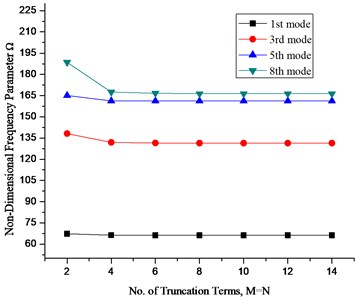It can be seen that the results converge very quickly even with small number of truncation terms. Thus a suitable truncation number should be used to achieve the accuracy of the largest desired frequency. In view of above and excellent convergence behavior of the current solution, the truncation number for subsequent calculation in the present method is taken as $M=N=$12.

After verifying the fast convergence of the preset method, results for Mindlin annular and circular plates and their sector counterparts are obtained and tabulated for various sector angles and different boundary conditions along with the reference results from literature. Table 3 shows fundamental frequency parameters for Mindlin annular sector plates having different sector angles and thickness to radius ratio. The plate has simply supported radial edges and different boundary conditions at the circular edges. The results have been compared with ABAQUS software as well as those available in literature.

Table 3Fundamental frequency parameter Ω=ωb2ρh/D1/2 for Mindlin annular sector plates having SS radial edges and various boundary conditions at the inner and outer circumferential edges (a/b= 0.5)

 Sector angle Ø $h/b$ Method Boundary conditions S-S S-F F-S F-C 195 0.1 Present 38.365 4.560 10.224 19.998 Ref  38.636 4.675 10.227 19.999 ABAQUS 38.580 4.540 11.159 20.923 0.2 Present 32.508 4.005 9.130 17.503 Ref  32.871 4.542 9.366 17.582 ABAQUS 32.676 4.067 10.014 18.239 210 0.1 Present 38.222 4.507 9.685 19.620 Ref  38.455 4.584 9.664 19.610 ABAQUS 38.223 4.230 9.479 19.516 0.2 Present 32.419 3.997 8.681 17.235 Ref  32.734 4.458 8.877 17.294 ABAQUS 32.469 3.923 8.590 17.201 270 0.1 Present 37.868 4.392 8.213 18.654 Ref  38.010 4.372 8.130 18.622 ABAQUS 37.875 4.197 8.015 18.574 0.2 Present 32.200 3.999 7.450 16.548 Ref  32.394 4.263 7.546 16.566 ABAQUS 32.252 3.932 7.366 16.524

Next we verify the applicability of this unified method for annular plates. As mentioned previously an annular plate can be viewed as a special case of annular sector plate if the sector angle becomes equal to 2$\pi$. Results for Mindlin annular plate for different combination of classical boundary conditions at the inner and outer edges for various cutout ratios are also calculated and presented in Table 4 along with those obtained from ABAQUS. A very close agreement can be observed in the calculated results. This close agreement verifies the applicability of the coupling spring technique for calculating frequency parameters for a complete annular plate without modifying the solution procedure.

Table 4Non dimensional frequency parameter Ω=ωb2ρh/D1/2 for Mindlin annular plates with various cutout ratio and boundary conditions (h/b= 0.2)

 B.C $a/b$ Method Mode sequence 1 2 3 4 5 SC 0.2 Present 21.161 22.228 22.228 27.545 27.550 ABAQUS 21.200 22.271 22.272 27.596 27.596 0.4 Present 32.154 32.829 32.829 35.411 35.414 ABAQUS 32.243 32.920 32.920 35.511 35.511 0.6 Present 56.120 56.458 56.458 57.642 57.643 ABAQUS 56.357 56.697 56.697 57.885 57.887 SF 0.2 Present 2.082 2.082 3.225 4.980 4.998 ABAQUS 2.084 2.084 3.224 4.975 4.975 0.4 Present 3.280 3.280 3.581 5.464 5.471 ABAQUS 3.282 3.282 3.578 5.465 5.465 0.6 Present 4.703 5.089 5.089 7.402 7.407 ABAQUS 4.699 5.090 5.090 7.411 7.411 FC 0.2 Present 9.476 16.774 16.774 26.240 26.247 ABAQUS 9.481 16.797 16.797 26.288 26.289 0.4 Present 12.156 15.995 15.995 24.240 24.246 ABAQUS 12.163 16.014 16.014 24.248 24.284 0.6 Present 21.219 22.812 22.812 27.275 27.278 ABAQUS 21.244 22.844 22.844 27.324 27.325 CF 0.2 Present 4.191 4.191 4.809 5.750 5.756 ABAQUS 4.193 4.193 4.810 5.746 5.748 0.4 Present 8.017 8.017 8.175 8.865 8.868 ABAQUS 8.022 8.022 8.179 8.870 8.870 0.6 Present 17.148 17.198 17.198 17.802 17.803 ABAQUS 17.168 17.220 17.220 17.826 17.826 CC 0.2 Present 24.346 25.313 25.313 29.515 29.519 ABAQUS 24.413 25.380 25.380 29.583 29.584 0.4 Present 37.641 38.196 38.196 40.238 40.240 ABAQUS 37.784 38.339 38.339 40.382 40.383 0.6 Present 64.159 64.462 64.462 65.485 65.486 ABAQUS 64.496 64.799 64.800 65.821 65.823

As mentioned earlier when the inner radius of an annular sector plate is approximated to a very small number say $a=$0.00001, then the annular sector plate converges to circular sector plate. The same method has been applied to circular sector plates and results for circular sector plates having different sector angles and boundary conditions at the radial and circumferential edges It should be noted that the symbol S stands for simply supported, C stands for clamped and F stand for free boundary conditions. The edges are taken in the counter clock wise direction, so SCS boundary conditions means simply supported radial edges and clamped circumferential edge. First three non-dimensional frequency parameters are calculated and presented in the Table 5 along with the reference results. It can be observed that the frequency parameters are in close agreement with the reference data.

Next we calculate the frequency parameter for various boundary conditions for a complete Mindlin circular plate having different thickness to radius ratio. In order to achieve this two simple modification needs to be done in the solution algorithm. First is equating the inner radius equal to a very small number say $a=$0.00001 and second is equating the sector angle equal to 2$\pi$ Table 6 presents first five non dimensional frequency parameter for a complete circular plate subjected to different boundary conditions at the circumferential edge and having different thickness to radius ratio. It should be noted that for the ‘F’; free boundary condition; the zero frequency parameters for the first six rigid body modes have not been taken into account in the Table 6. It can be observed that the frequency parameter decreases with increasing thickness to radius ratio in all the three types of boundary conditions listed. A good agreement between the presented results and those obtained through ABAQUS can also be observed which proves the applicability of the present method for calculating the frequency parameters for Mindlin circular plates also.

Table 5First three non-dimensional frequency parameters Ω=ωb2ρh/D1/2 for circular sector plates having different combination of classical boundary conditions and sector angle (h/b= 0.2, a/b= 0.00001)

 Sector angle Ø BC Mode sequence Present Ref.  ABAQUS 30 SCS 1 66.256 67.933 66.490 2 98.936 102.560 99.373 3 131.364 132.860 132.146 90 SSS 1 21.006 21.977 21.030 2 41.254 42.699 41.339 3 48.863 50.307 48.981 120 CCC 1 27.311 27.314 27.372 2 40.977 40.983 41.105 3 52.324 52.338 52.515

Table 6First five non-dimensional frequency parameter Ω=ωb2ρh/D1/2 for a Mindlin circular plate having different boundary conditions and thickness to radius ratio (a/b= 0.00001)

 B.C $h/b$ Method Mode Sequence 1 2 3 4 5 C 0.1 Present 9.941 20.178 20.178 32.210 32.223 ABAQUS 9.939 20.176 20.176 32.220 32.222 0.2 Present 9.240 17.758 17.758 26.994 27.000 ABAQUS 9.246 17.782 17.782 27.044 27.045 0.25 Present 8.807 16.446 16.446 24.478 24.482 ABAQUS 8.816 16.479 16.479 24.540 24.540 S 0.1 Present 4.895 13.512 13.512 24.324 24.336 ABAQUS 4.892 13.508 13.508 24.315 24.317 0.2 Present 4.777 12.620 12.620 21.690 21.696 ABAQUS 4.776 12.625 12.625 21.710 21.710 0.25 Present 4.696 12.080 12.080 20.272 20.276 ABAQUS 4.696 12.089 12.089 20.300 20.300 F 0.1 Present 5.283 5.299 8.869 12.153 12.248 ABAQUS 5.275 5.275 8.865 12.062 12.062 0.2 Present 5.117 5.125 8.505 11.366 11.428 ABAQUS 5.113 5.113 8.504 11.316 11.316 0.25 Present 5.011 5.018 8.268 10.910 10.960 ABAQUS 5.009 5.009 8.268 10.871 10.871

All the results tabulated so far have been calculated for various combinations of classical boundary conditions which are treated as a special case of elastic boundary conditions in which the stiffness values for the restraining springs are set either equal to a very high value i.e. 1014 or a very low number zero. It is therefore necessary to study the effect of these restraining spring stiffnesses on the frequency characteristics for these plates. Figs. 4-6 shows the effect of boundary restraining springs on the frequency parameter ‘$\mathrm{\Omega }$’ for a fully clamped annular plate having $a/b=$ 0.6 and $h/b=$ 0.2.

Fig. 4 shows effect of translational spring stiffness on the second and sixth mode frequency parameter of annular plate in which the stiffness of the translational spring stiffness varies from 0 to 1e14 while the stiffnesses of the rotational spring in radial and tangential direction ( ) are kept constant i.e. 1e14.

Similarly, Figs. 5 and 6 have been obtained by assigning the corresponding boundary spring stiffness, a value ranging from 0 to 1014 and keeping the stiffnesses of other sets of spring equal to 1014.

Fig. 4Effect of translational spring stiffness k on frequency parameter Ω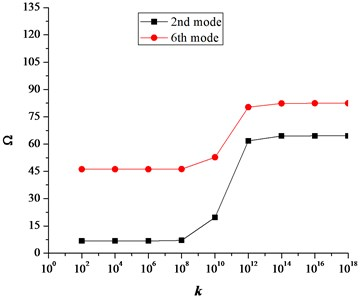Fig. 5Effect of rotational spring stiffness K attached in tangential direction on Ω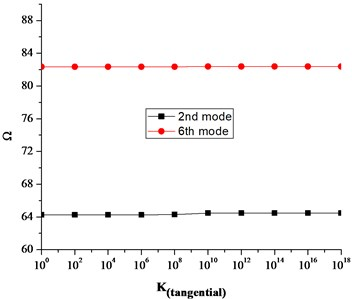Fig. 6Effect of rotational spring stiffness K attached in radial direction on Ω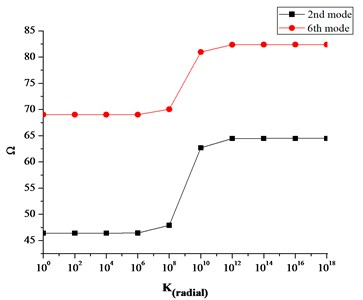Similarly, Fig. 7(a)-(c) shows the effect of coupling springs on the frequency parameter $\mathrm{\Omega }$.

It can be seen that the translational and rotational boundary springs sufficiently affect the frequency parameters. More precisely the translational boundary restraining spring tend to be more influential when its stiffness varies from 108 to 1013. Similarly, the influential range for the rotational boundary spring in the radial direction is 106 to 1012. However, the influence of rotational boundary spring in the tangential direction is very small as seen in Fig. 5. Also it can be seen in Fig. 7(a)-(c) that influential range for the coupling springs is much smaller as compared to the boundary restraining springs. This influential range is the elastic range and frequency parameters can easily be calculated for elastic boundary conditions by assigning the proper stiffness values to the boundary restraining springs without modifying the solution procedure or algorithms.

We know that in practical engineering, designing or development of any mechanical system or a product, structure vibration analysis and testing is an important part to assess the real behavior of the structure when subjected to static or dynamic loads. In other words, to better understand any structural vibration problem, the resonant frequencies of a structure need to be identified and quantified in order to avoid well known resonance phenomena which can result in catastrophe. Today, modal analysis has become a widespread means of finding the modes of vibration of a machine or a structure.

Fig. 7Effect of coupling springs on the frequency parameter Ω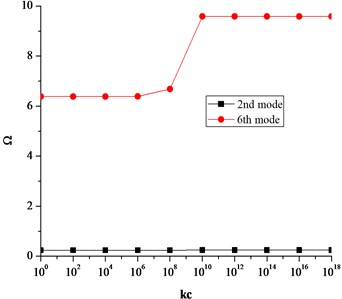a) Effect of translational coupling spring $kc$ on frequency parameter $\mathrm{\Omega }$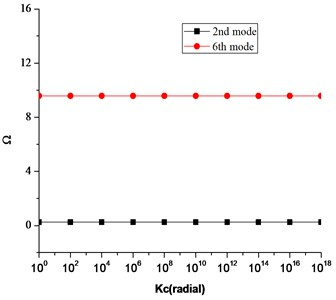b) Effect of rotational coupling spring in radial direction $Kc$ on frequency parameter $\mathrm{\Omega }$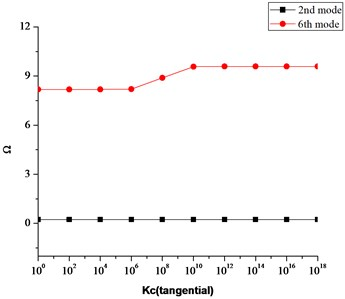c) Effect of rotational coupling spring in tangential direction $Kc$ on frequency parameter $\mathrm{\Omega }$

Various analytical methods have been developed over the years to accurately estimate the resonant frequencies or modes of vibrations of any structure when subjected to different boundary conditions. Once these frequencies are calculated they are used to estimate the modes of vibrations of a structure which are determined by the material properties and boundary conditions. Each mode of vibration is defined by a natural (modal or resonant) frequency, modal damping, and a mode shape. If there is a slight change in material properties or boundary conditions of a structure, its modes of vibration will also change. Therefore, it is important to estimate these frequencies for any change in material properties as well as boundary conditions because in practical engineering applications, the material properties of a structure and boundary conditions may vary. Furthermore, most of the existing techniques available so far to estimate these natural or resonant frequencies are limited to classical boundary conditions (clamped, free, simply supported etc.), however in practical engineering applications the structures are not always subjected to classical boundary conditions rather they may be subjected to elastic boundary conditions.

In the present manuscript, the unified method presented not only helps to accurately estimate these natural frequencies of circular and annular plates and their sector counter parts when they are subjected to classical boundary conditions but also when they are subjected to general elastic boundary conditions. The presented results give an insight of the modes of vibration of these plates having different material properties and subjected to elastic boundary conditions. Moreover, another important contribution of this technique is that this method does not require any changes in procedure or solution algorithms to accommodate different geometries, material properties or boundary conditions. The same solution algorithm or procedure can be used to estimate natural frequencies for different materials and boundary conditions. Different boundary conditions (classical, elastic, uniform & non-uniform) can easily be achieved by simply changing the stiffnesses of the translational and rotational springs attached at the boundaries or edges of these plates”.

## 4. Conclusion

In this paper a unified method is presented for vibration analysis of Mindlin annular, circular and their sector counter parts with arbitrary boundary conditions at their edges. Coupling springs technique has been utilized to avoid inconvenient formulation or procedural modification to accommodate different boundary conditions and geometrical shapes of the plates. Irrespective of the shape of the plate and the type of boundary conditions, each of the displacement function is expressed as a new form of trigonometric expansion with high convergence rate. Rayleigh-Ritz method has been used to determine the expansion coefficients. The current method therefore can be universally applied to a wide range of vibration problems involving different shapes, boundary conditions, varying materials and geometric properties without modifying the solution algorithms and procedure. The unification, fast convergence, accuracy and reliability have been fully demonstrated through several numerical examples involving different shapes and boundary conditions. Furthermore, the effect of boundary restraining springs and coupling springs on the frequency parameter have also been studied.

#### Cited by

Symmetric and asymmetric vibrations of rotating GPLRC annular plate

14 January 2016
Accepted
17 August 2016
Published
31 December 2016
SUBJECTS
Mechanical vibrations and applications
Keywords
vibrations
circular plates
annular plates
sector plates
natural frequency
mode shapes
arbitrary boundary conditions
Acknowledgements

The authors gratefully acknowledge the financial support from the National Natural Science Foundation of China (No. U1430236) and Natural Science Foundation of Heilongjiang Province of China (No. E2016024)